# Simple Vectors Worksheet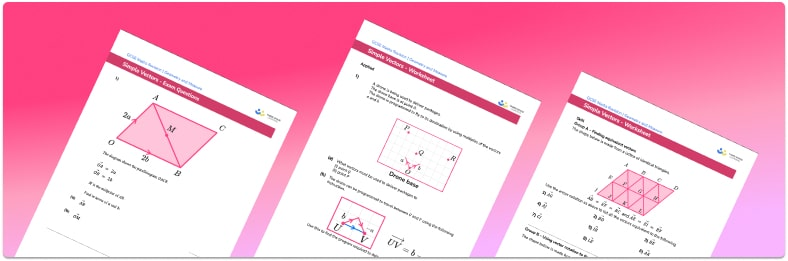• Section 1 of the simple vectors worksheet contains 20+ skills-based simple vectors questions, in 3 groups to support differentiation
• Section 2 contains 4 applied simple vectors questions with a mix of worded problems and deeper problem solving questions
• Section 3 contains 4 foundation and higher level GCSE exam style simple vectors questions
• Answers and a mark scheme for all simple vectors questions are provided
• Questions follow variation theory with plenty of opportunities for students to work independently at their own level
• All questions created by fully qualified expert secondary maths teachers
• Suitable for GCSE maths revision for AQA, OCR and Edexcel exam boards

• This field is for validation purposes and should be left unchanged.

You can unsubscribe at any time (each email we send will contain an easy way to unsubscribe). To find out more about how we use your data, see our privacy policy.

### Simple vectors at a glance

A vector describes a movement. A vector has both a magnitude and a direction. The magnitude of a vector could be described as the size of the vector, or how long the vector is.

The vector describing the movement from A to B can be denoted in three different ways: a capital AB with an arrow pointing to the right written above it, a, or a

The reverse vector moves in the opposite direction. Here, the reverse vector from A to B would be written as a capital BA with an arrow pointing to the right written above it, –a, or –a

Vectors (vector quantities) can also be described using a column vector.

For example, in the parallelogram ABCD, a can represent the vector that moves you from vertex A to vertex B. The sides AB and CD are parallel and have equal length. To get from vertex D to vertex C, we would label this with the same vector, a. If we start at vertex C and travel along the reverse vector –a, we will get to vertex D.

A scalar is a quantity that has magnitude (a size) but no direction. Vectors can be multiplied by scalar quantities to make them shorter, longer or change their direction. This is called scalar multiplication. For example, the vector 2a is twice as long as the vector a. Vector problems may involve travelling part way along line segments, for example to the midpoint of a line. This can be done using scalar multiplication.

We can use vector addition and subtraction to combine vectors and find a pathway from one point to another. The overall movement is known as a resultant vector. For example, if we move along a and then along b, the resultant vector would be a+b. This can be used to construct 2-dimensional shapes such as a regular hexagon.

In GCSE maths, we will only consider vectors in 2d space, but they can be used in 3d space to describe the movement around solids such as a cube or tetrahedron.

Looking forward, students can then progress to additional geometry worksheets, for example the vector problems worksheet, and the column vectors worksheet.For more teaching and learning support on Geometry our GCSE maths lessons provide step by step support for all GCSE maths concepts.

## Related worksheets

Vector Problems Worksheet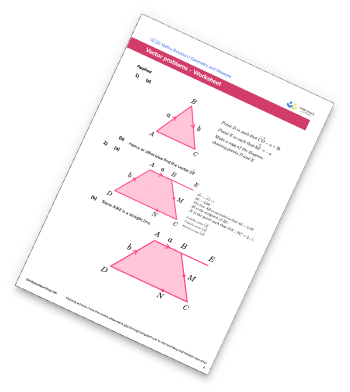Vectors Worksheet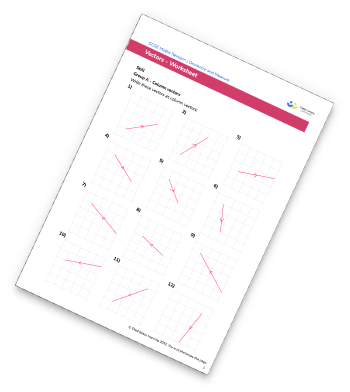Congruent Triangles Worksheet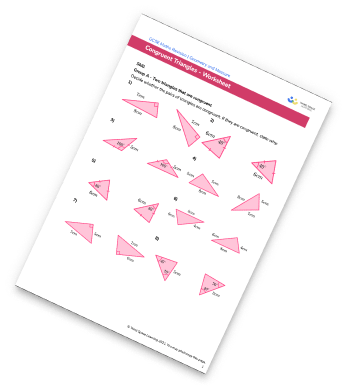Volume Worksheet – How To Calculate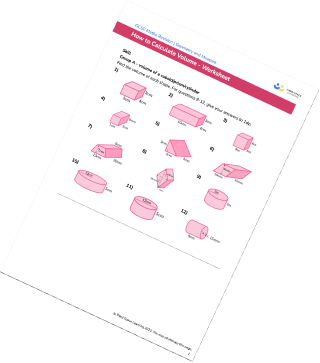## Do you have KS4 students who need more focused attention to succeed at GCSE?There will be students in your class who require individual attention to help them succeed in their maths GCSEs. In a class of 30, it’s not always easy to provide.

Help your students feel confident with exam-style questions and the strategies they’ll need to answer them correctly with our dedicated GCSE maths revision programme.

Lessons are selected to provide support where each student needs it most, and specially-trained GCSE maths tutors adapt the pitch and pace of each lesson. This ensures a personalised revision programme that raises grades and boosts confidence.

Find out more